## ML Aggarwal Class 8 Solutions for ICSE Maths Chapter 17 Visualising Solid Shapes Objective Type Questions

Mental Maths
Question 1.
Fill in the blanks:
(i) A solid made up of polygonal regions is called ………
(ii) Polyhedrons which are not convex are called ………
(iii) A polyhedron is said to be ……… if all of its faces are regular polygons and the same number of faces meet at each vertex.
(iv) A polyhedron whose base and top are congruent polygons and whose lateral faces are parallelograms in shape is called a ………
(v) A prism whose base and top are congruent hexagon is called a ………
(vi) A polyhedron whose all lateral faces are triangles is called a ………
(vii) A triangular pyramid is also known as ………
(viii) A rectangular prism is also known as ………
(ix) The polygonal regions forming a polyhedron are called ………
Solution:
(i) A solid made up of polygonal regions is called polyhedron.
(ii) Polyhedrons which are not convex are called concave polyhedron.
(iii) A polyhedron is said to be regular polyhedron if all of its faces are
regular polygons and the same number of faces meet at each vertex.
(iv) A polyhedron whose base and top are congruent polygons and
whose lateral faces are parallelograms in shape is called a prism.
(v) A prism whose base and top are congruent hexagon is called a hexagonal prism.
(vi) A polyhedron whose all lateral faces are triangles is called a triangular pyramid.
(vii) A triangular pyramid is also known as a tetrahedron.
(viii) A rectangular prism is also known as a cuboid.
(ix) The polygonal regions forming a polyhedron are called faces.

Question 2.
State which of the following statements are true (T) or false (F):
(i) A cylinder is a polyhedron.
(ii) All the prisms and pyramids are polyhedrons.
(iii) A tetrahedron is the only pyramid which can be a regular polyhedron.
(iv) The line segments where the faces of a polyhedron meet are called edges.
(v) F + E = V + 2 is called a Euler’s formula.
(vi) In any prism number of faces is 2 more than number of sides of polygonal base.
(vii) In any pyramid number of edges is twice the number of sides of polygonal base.
(viii) An octagonal prism has 18 vertices.
(ix) All pyramids are prisms.
(x) Lateral faces of a pyramid are triangles.
Solution:
(i) A cylinder is a polyhedron. False
(ii) All the prisms and pyramids are polyhedrons. True
(iii) A tetrahedron is the only pyramid
which can be a regular polyhedron. True
(iv) The line segments where the faces of
a polyhedron meet are called edges. True
(v) F + E = V + 2 is called a Euler’s formula. False
Correct:
It is F + V = E + 2.
(vi) In any prism number of faces is 2 more than
the number of sides of polygonal base. True
(vii) In any pyramid number of edges is twice
the number of sides of polygonal base. True
(viii) An octagonal prism has 18 vertices. False Correct:
It has 8 + 8 = 16
(ix) All pyramids are prisms. False
Correct:
Both are different shapes.
(x) Lateral faces of a pyramid are triangles. True

Multiple Choice Questions
Choose the correct answer from the given four options (3 to 17):
Question 3.
Which of the following is not a 2-dimensional shape?
(a) Triangle
(b) Circle
(c) Sphere
(d) Rectangle
Solution:
Sphere is not a two-dimensional shape, (c)

Question 4.
Which of the following is a 3-dimensional shape?
(a) Parallelogram
(b) Cylinder
(c) Square
(d) none of these
Solution:
Cylinder is a 3-dimensional shape. (b)

Question 5.
Name the pyramid shown in the given figure.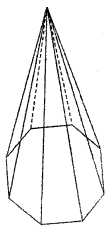(a) Nonagonal pyramid
(b) Octagonal pyramid
(c) Decagonal pyramid
(d) Hexagonal pyramid
Solution:
Octagonal pyramid. (b)

Question 6.
How many parallel and congruent faces does a cylinder have?
(a) 4
(b) 3
(c) 2
(d) none
Solution:
A cylinder has two parallel and congruent faces. (c)

Question 7.
How many pairs of congruent parallel faces does a rectangular prism have?
(a) 8
(b) 6
(c) 4
(d) 3
Solution:
A rectangular prism has 3 pairs of congruent
and parallel faces. (d)

Question 8.
How many congruent isosceles triangle does a square pyramid have?
(a) 2
(b) 4
(c) 6
(d) 8
Solution:
A square pyramid has 4 congruent isosceles triangles. (b)

Question 9.
Which amongst the following is not a polyhedron?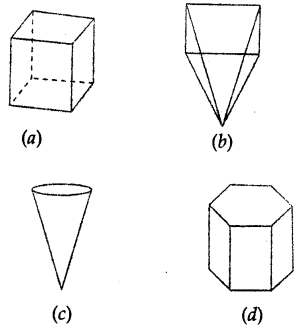Solution:
Figure (c) is not a polyhedron. (c)

Question 10.
Which of the following is not a prism?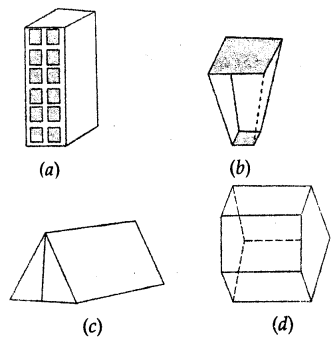Solution:
Figure (b) is not a prism. (b)

Question 11.
The number of triangular faces of a triangular prism are
(a) 2
(b) 3
(c) 4
(d) 5
Solution:
A triangular prism has 2 triangular faces, (a)

Question 12.
The number of edges in a pentagonal pyramid are
(a) 5
(b) 10
(c) 15
(d) 20
Solution:
A pentagonal pyramid has 10 edges. (b)

Question 13.
The number of rectangular faces in a hexagonal prism are
(a) 6
(b) 8
(c) 10
(d) 12
Solution:
A hexagonal prism has 6 rectangular faces. (a)

Question 14.
In a polyhedron E = 15, V = 10, then F is
(a) 3
(b) 5
(c) 7
(d) 9
Solution:
E = 15, V = 10
F + V = E + 2
⇒ F = E + 2 – V = 15 + 2 – 10 = 7 (c)

Question 15.
In a polyhedron F = 5, E = 8, then V is
(a) 3
(b) 5
(c) 7
(d) 9
Solution:
F + V = E + 2
⇒ 5 + V = 8 + 2
⇒ 5 + V = 10
⇒ V = 10 – 5 = 5 (b)

Question 16.
In a polyhedron F = 17, V = 30, then E is
(a) 30
(b) 45
(c) 60
(d) none of these
Solution:
F = 17, V = 30
F + V = E + 2
⇒ E = F + V – 2
⇒ E = 17 + 30 – 2 = 45 (b)

Question 17.
A polyhedron have 4 faces, 4 vertices and 6 edges. Name the polyhedron.
(a) A rectangular prism
(b) A triangular prism
(c) A rectangular pyramid
(d) A triangular pyramid
Solution:
A polyhedron has 4 faces, 4 vertices and 6 edges.
∴ It is triangular pyramid. (d)

Higher Order Thinking Skills (Hots)
Question 1.
Which of the following nets can be folded to form a cone?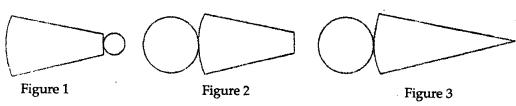Solution:
Net in figure (3) can be folded to form a cone.

Question 2.
Which of the following nets can be folded to form a cylinder?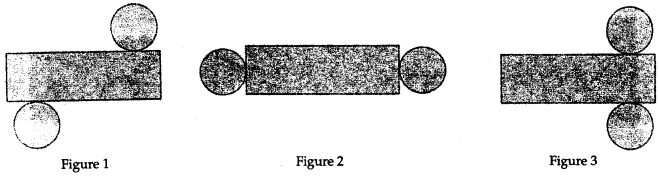Solution:
Net in the figure (1) and (2) can be folded to form a cylinder.